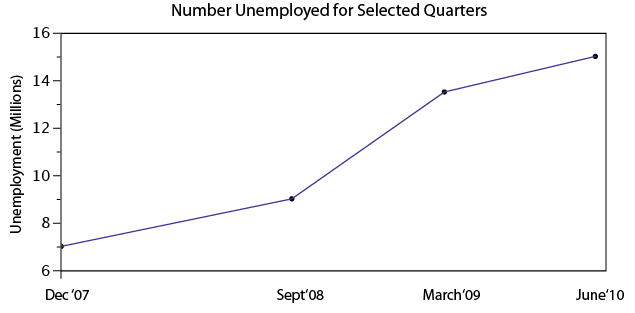# 4.11: Statistical Literacy

•• David Lane
• Rice University
$$\newcommand{\vecs}{\overset { \rightharpoonup} {\mathbf{#1}} }$$ $$\newcommand{\vecd}{\overset{-\!-\!\rightharpoonup}{\vphantom{a}\smash {#1}}}$$$$\newcommand{\id}{\mathrm{id}}$$ $$\newcommand{\Span}{\mathrm{span}}$$ $$\newcommand{\kernel}{\mathrm{null}\,}$$ $$\newcommand{\range}{\mathrm{range}\,}$$ $$\newcommand{\RealPart}{\mathrm{Re}}$$ $$\newcommand{\ImaginaryPart}{\mathrm{Im}}$$ $$\newcommand{\Argument}{\mathrm{Arg}}$$ $$\newcommand{\norm}{\| #1 \|}$$ $$\newcommand{\inner}{\langle #1, #2 \rangle}$$ $$\newcommand{\Span}{\mathrm{span}}$$ $$\newcommand{\id}{\mathrm{id}}$$ $$\newcommand{\Span}{\mathrm{span}}$$ $$\newcommand{\kernel}{\mathrm{null}\,}$$ $$\newcommand{\range}{\mathrm{range}\,}$$ $$\newcommand{\RealPart}{\mathrm{Re}}$$ $$\newcommand{\ImaginaryPart}{\mathrm{Im}}$$ $$\newcommand{\Argument}{\mathrm{Arg}}$$ $$\newcommand{\norm}{\| #1 \|}$$ $$\newcommand{\inner}{\langle #1, #2 \rangle}$$ $$\newcommand{\Span}{\mathrm{span}}$$$$\newcommand{\AA}{\unicode[.8,0]{x212B}}$$

## Are Commercial Vehicles in Texas Unsafe?

Prerequisites

Graphing Distributions

A news report on the safety of commercial vehicles in Texas stated that one out of five commercial vehicles have been pulled off the road in $$2012$$ because they were unsafe. In addition, $$12,301$$ commercial drivers have been banned from the road for safety violations.

The author presents the bar chart below to provide information about the percentage of fatal crashes involving commercial vehicles in Texas since $$2006$$. The author also quotes DPS director Steven McCraw:

Commercial vehicles are responsible for approximately $$15$$ percent of the fatalities in Texas crashes. Those who choose to drive unsafe commercial vehicles or drive a commercial vehicle unsafely pose a serious threat to the motoring public.

Example $$\PageIndex{1}$$

Based on what you have learned in this chapter, does this bar chart below provide enough information to conclude that unsafe or unsafely driven commercial vehicles pose a serious threat to the motoring public? What might you conclude if $$30$$ percent of all the vehicles on the roads of Texas in $$2010$$ were commercial and accounted for $$16$$ percent of fatal crashes?Figure $$\PageIndex{1}$$: Crash Statistics for commercial vehicles in Texas

Solution:

This bar chart does not provide enough information to draw such a conclusion because we don’t know, on the average, in a given year what percentage of all vehicles on the road are commercial vehicles. For example, if $$30$$ percent of all the vehicles on the roads of Texas in $$2010$$ are commercial ones and only $$16$$ percent of fatal crashes involved commercial vehicles, then commercial vehicles are safer than non-commercial ones. Note that in this case $$70$$ percent of vehicles are non-commercial and they are responsible for $$84$$ percent of the fatal crashes.

## Linear By Design

Example $$\PageIndex{2}$$

Fox News aired the line graph below showing the number unemployed during four quarters between $$2007$$ and $$2010$$.Figure $$\PageIndex{2}$$: Fox news graph showing job loss by quarter

Does Fox News' line graph provide misleading information? Why or Why not?

Solution:

There are major flaws with the Fox News graph. First, the title of the graph is misleading. Although the data show the number unemployed, Fox News’ graph is titled "Job Loss by Quarter." Second, the intervals on the $$X$$-axis are misleading. Although there are $$6$$ months between September $$2008$$ and March $$2009$$ and $$15$$ months between March $$2009$$ and June $$2010$$, the intervals are represented in the graph by very similar lengths. This gives the false impression that unemployment increased steadily.

The graph presented below is corrected so that distances on the $$X$$-axis are proportional to the number of days between the dates. This graph shows clearly that the rate of increase in the number unemployed is greater between September $$2008$$ and March $$2009$$ than it is between March $$2009$$ and June $$2010$$.Figure $$\PageIndex{3}$$: Corrected Fox News graph

## Contributors

This page titled 4.11: Statistical Literacy is shared under a Public Domain license and was authored, remixed, and/or curated by David Lane.